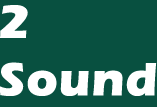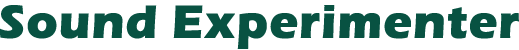If you've wanted more control over your Atari's sound, here's a solution. You can use this program to experiment, to add sound to other programs (via the SOUND or POKE instructions), and to govern all four voices and aspects of special effects.

Sound is one of the most important capabilities of the Atari computer. Not only does it permit four-part harmony if you are so inclined, but sound is an essential ingredient in games. It transports you into the world of the game, filling your ears with the sound of a laser cannon, letting you hear force shields as they collapse around you.

Unfortunately, the sound commands are among the most difficult to experiment with. The SOUND instruction can sometimes be clumsy and inconvenient; for one thing, the sounds stay on until you turn them off with another SOUND instruction. Also, you can't achieve the full range of sound with the BASIC instruction, since using it changes any settings in AUDCTL (the register which controls sound effects).

Sound control is a complicated matter, and simple programs cannot offer you complete control over the sounds. Joysticks couldn't govern four channels with nine registers.

This program takes a little practice to get used to, but it permits total control over all sound registers plus AUDCTL, turns the channels on individually, and shuts them all off at once when you need silence. When you are satisfied with the sounds, you can display the appropriate BASIC statements in either the POKE or the SOUND format.

An Overview

Lets first briefly summarize the Atari sound system. (For complete details, see the Atari Personal Computer System Hardware Manual, pages III.12 through III.14.) There are four independent sound channels whose distortion, frequency, and volume can be independently controlled. These are addressed by the SOUND instruction with the numbers 0 through 3. The Hardware Manual refers to them as 1 through 4. The sound datea can be independently POKEd into registers 53760 though 53767. The odd numbers control volume and distortion, and the even numbers control the frequency. Register 53768 is AUDCTL, which controls all of the sound channels in one way or another. If you use the BASIC SOUND instruction, any changes you may have made to AUDCTL are reset—AUDCTL is set to zero. Thus you do not have full control of the sounds with the SOUND instruction.

This program attempts to give you easy control over all of these parameters. Compromises to reduce complexity have been made in favor of the notation and numbers used in the SOUND instruction. See the BASIC Reference Manual for further information.

The figure shows the display that you will see upon RUNning and entering the commands. The first eight lines, numbered B7 through B0, are the bits in the AUDCTL Register. To change bit seven to 1, type B7 and RETURN. To change it back to zero, type B7 and RETURN again. These are technical changes that give no indication of what the new sound will be like. Experimentation is best. Suffice it to say that using B1 through B4 turns on both of the sound channels associated with bit seven.

To discuss the next five lines of the figure, we have to jump down to the lines labeled D: and X:. There are two types of entriesto make this program, those which are purely commands and those which require numbers. If you need to enter a number, enter the number first and press RETURN. If it is a pure command, simply enter the command and RETURN. If you wish to work with sound channel zero, type the following sequence: 0, RETURN, REG, RETURN. A 0 will appear after SOUND (REG)ISTER on the display. For a pure tone, type 10, RETURN, DIS, RETURN, and a 10 will appear after (DIS)TORTION:. Similarly, 100, RETURN, FRE, RETURN, and 8, RETURN, VOL, RETURN, will complete this part of the display.

To hear this sound, type 0, RETURN, CH, RETURN, and to turn it off, type OFF, RETURN. To see the POKE values for this sound, type PDIS, RETURN, and the list of nine POKEs will appear on the screen. Copy these POKEs into your program, and you will duplicate the sound that you hear. The top right POKE is AUDCTL. The next four rows are channels 0 through 3—the left column is the distortion and the volume, and the right one is the frequency for each channel.

If AUDCTL is0—which is the same as bits B0 through B7 being all 0—then the SOUND instruction may be used. To see the SOUND instructions, type SDIS, RETURN, and the POKEs will be replaced with SOUNDs.

The "force" output is in the odd-numbered POKE registers and produces a click from the TV. It is turned off and on by the use of FRC, RETURN. If you have set any of the AUDCTL bits, you must use the POKEs to duplicate the sounds. The sound channels must be turned on individually by the CH command. OFF turns off all channels. If you make a change and want to hear it, type the channel number and CH again. This may seem cumbersome, but otherwise the sounds would always be on.

```Screen Display
AUDCTL (REG)ISTER 4
9 BIT POLY:(B7): 0
clock Ch.0 w/1.79 MHz:(B6): 0
clock Ch.2 w/1.79 MHz:(B5): 0
clock Ch.1 w/Ch.0:(B4): 0
clock Ch.3 w/Ch.2:(B3): 0
clock Ch.0 w/Ch.2 HiP:(B2): 0
clock Ch.1 w/Ch.3 HiP:(B1): 0
15 kHz:(B0): 0
SOUND (REG)ISTER 0
(DIS)TORTION:10
(FRE)QUENCY:100
FORCE OUTPUT:0
(VOL)UME:8
X:
D: ?
REG DIS FRE FRC VOL
OFF CH
PDIS SDIS         POKE 53768, 0
POKE 53761, 168   POKE 53760, 100
POKE 53763, 0     POKE 53762, 0
POKE 53765, 0     POKE 53764, 0
POKE 53767, 0     POKE 53766, 0
```

Sound Experimenter
```80 DIM S(5,8),IN\$(50)
90 FOR I=0 TO 8:FOR J=0 TO 5:S(J,I)=0:NEXT J:NEXT I
100 REG=5000:DIS=5100:FRE=5200:FRC=5300:OFF=5400
102 CLD=5900:CLX=6000:VOL=6100:POKAUD=6200:CH=6300:START=6400:REGDIS=6500:BUZZ=6600
104 PDIS=6700:SDIS=6800:EDIS=6900
1000 REM DISPLAY
1002 GRAPHICS 0:POKE 751,1
1008 POSITION 2,0:? "AUDCTL (REG)ISTER 4"
1010 POSITION 2,1:? "{11 SPACES}9 BIT POLY:(B7):"
1020 POSITION 2,2:? "clock CH.0 w/1.79 MHz:(B6):"
1030 POSITION 2,3:? "clock CH.2 w/1.79 MHz:(B5):"
1040 POSITION 2,4:? "{4 SPACES}clock Ch.1 w/Ch.0:(B4):"
1050 POSITION 2,5:? "{4 SPACES}clock Ch.3 w/Ch.2:(B3):"
1060 POSITION 2,6:? "clock Ch.0 w/Ch.2 HiP:(B2):"
1070 POSITION 2,7:? "clock Ch.1 w/Ch.3 HiP:(B2):"
1080 POSITION 2,8:? "{15 SPACES}15 kHz:(B0):"
1090 POSITION 2,9:? "{5 SPACES}SOUND (REG)ISTER"
1100 POSITION 2,10:? "{6 SPACES}(DIS)TORTION:"
1110 POSITION 2,11:? "{7 SPACES}(FRE)QUENCY:"
1120 POSITION 2,12:? "{6 SPACES}FORCE OUTPUT:"
1126 POSITION 2,13:? "{10 SPACES}(VOL)UME:"
1128 POSITION 2,14:? "X:"
1130 POSITION 2,15:? "D:"
1140 POSITION 2,16:? "REG DIS FRE FRC VOL"
1150 POSITION 2,17:? "OFF CH"
1160 POSITION 2,18:? "PDIS SDIS"
1500 GOSUB START
2000 REM JUMP TABLE
2008 FOR ZZZ=1 TO 2 STEP 0
2010 POSITION 5,15:POKE 752,0:INPUT IN\$:POKE 752,1
2020 TRAP 2040:A=VAL(IN\$):TRAP 40000
2030 POSITION 5,14:? A:GOSUB CLD
2040 IF IN\$="REG" THEN GOSUB REG
2042 IF IN\$="DIS" THEN GOSUB DIS
2044 IF IN\$="FRE" THEN GOSUB FRE
2046 IF IN\$="FRC" THEN GOSUB FRC
2048 IF IN\$="OFF" THEN GOSUB OFF
2049 IF IN\$="CH" THEN GOSUB CH
2058 IF IN\$="VOL" THEN GOSUB VOL
2060 IF IN\$="B7" THEN S(4,7)= NOT (S(4,7)):POSITION 30,1:? S(4,7):GOSUB CLD
2061 IF IN\$="B6" THEN S(4,6)= NOT (S(4,6)):POSITION 30,2:? S(4,7):GOSUB CLD
2062 IF IN\$="B5" THEN S(4,5)= NOT (S(4,5)):POSITION 30,3:? S(4,7):GOSUB CLD
2063 IF IN\$="B4" THEN S(4,4)= NOT (S(4,4)):POSITION 30,4:? S(4,7):GOSUB CLD
2064 IF IN\$="B3" THEN S(4,3)= NOT (S(4,3)):POSITION 30,5:? S(4,7):GOSUB CLD
2065 IF IN\$="B2" THEN S(4,2)= NOT (S(4,2)):POSITION 30,6:? S(4,7):GOSUB CLD
2066 IF IN\$="B1" THEN S(4,1)= NOT (S(4,1)):POSITION 30,7:? S(4,7):GOSUB CLD
2067 IF IN\$="B0" THEN S(4,0)= NOT (S(4,0)):POSITION 30,8:? S(4,7):GOSUB CLD
2070 IF IN\$="PDIS" THEN GOSUB PDIS
2072 IF IN\$="SDIS" THEN GOSUB SDIS
2980 IF FAIL=1 THEN GOSUB BUZZ
2989 FAIL=0
2990 NEXT ZZZ
5000 REM REG REGISTER SET
5010 IF A<0 OR A>3 THEN FAIL=1
5020 IF A>0 OR A<4 THEN POSITION 24,9:? A
5030 C=A:REM S(C,B)
5040 GOSUB REGDIS
5088 GOSUB CLD:GOSUB CLX
5090 RETURN
5100 REM DIS DISTORTION LEVEL
5110 IF A<0 OR A>14 THEN FAIL=1:GOTO 5180
5112 IF INT(A/2)-A/2<>0 THEN FAIL=1:GOTO 5180
5120 IF A=0 THEN D1=0
5121 IF A=2 THEN D1=32
5122 IF A=4 THEN D1=64
5123 IF A=6 THEN D1=96
5124 IF A=8 THEN D1=128
5125 IF A=10 THEN D1=160
5126 IF A=12 THEN D1=192
5127 IF A=14 THEN D1=224
5130 POSITION 21,10:? A
5140 S(C,1)=D1:S(C,5)=A
5170 S(C,8)=A
5180 GOSUB CLD:GOSUB CLX
5190 RETURN
5200 REM FRE FREQUENCY STORE
5210 IF A<O OR A>255 THEN FAIL=1
5218 POSITION 21,11:? "{8 SPACES}"
5220 POSITION 21,11:? A
5230 S(C,2)=A
5280 GOSUB CLD:GOSUB CLX
5290 RETURN
5300 REM FRC  SET FORCE BIT
5310 IF A=0 THEN S(0,3)= NOT S(0,3)
5320 IF A=0 THEN S(1,3)= NOT S(1,3)
5330 IF A=0 THEN S(2,3)= NOT S(2,3)
5340 IF A=0 THEN S(3,3)= NOT S(3,3)
5350 POSITION 21,12:? S(C,3)
5380 GOSUB CLD
5390 RETURN
5400 REM OFF TURN OFF SOUND
5410 POKE 53761,0:POKE 53763,0:POKE 53765,0:POKE 53767,0
5480 GOSUB CLD
5490 RETURN
5900 REM CLD CLEAR D POS.
5910 POSITION 5,15:? "{20 SPACES}"
5990 RETURN
6000 REM CLX CLEAR X POS.
6010 POSITION 5,14:? "{21 SPACES}":A=0
6090 RETURN
6100 REM VOL VOLUME SET
6110 IF A<0 OR A>15 THEN FAIL=1:GOTO 6180
6120 POSITION 21,13:? "{12 SPACES}"
6122 POSITION 21,13:? A
6130 S(C,4)=A
6180 GOSUB CLD:GOSUB CLX
6190 RETURN
6200 REM POKAUD POKE AUDCTL VALUE
6208 SUM=0
6210 IF S(4,0)=1 THEN SUM=SUM+1
6211 IF S(4,1)=1 THEN SUM=SUM+2
6212 IF S(4,2)=1 THEN SUM=SUM+4
6213 IF S(4,3)=1 THEN SUM=SUM+8
6214 IF S(4,4)=1 THEN SUM=SUM+16
6215 IF S(4,5)=1 THEN SUM=SUM+32
6216 IF S(4,6)=1 THEN SUM=SUM+64
6217 IF S(4,7)=1 THEN SUM=SUM+128
6220 POKE 53768,SUM
6290 RETURN
6300 REM CH TURN ON SOUND CHANNELS
6310 GOSUB POKAUD
6320 IF A=0 THEN POKE 53761,S(0,1)+S(0,4):POKE 53760,S(0,2)
6322 IF A=1 THEN POKE 53763,S(1,1)+S(1,4):POKE 53762,S(1,2)
6324 IF A=2 THEN POKE 53765,S(2,1)+S(2,4):POKE 53764,S(2,2)
6326 IF A=3 THEN POKE 53767,S(3,1)+S(3,4):POKE 53766,S(3,2)
6380 GOSUB CLX:GOSUB CLD:GOSUB REGDIS
6390 RETURN
6400 REM START  SET UP
6410 FOR I=1 TO 8:POSITION 30,I:? "0":NEXT I
6490 RETURN
6500 REM REGDIS  DISPLAY OF REGISTER
6505 POSITION 21,12:? "{3 SPACES}"
6506 POSITION 21,12:? S(C,3)
6510 POSITION 21,11:? "{6 SPACES}"
6511 POSITION 21,11:? S(C,2)
6520 POSITION 21,10:? "{6 SPACES}"
6521 POSITION 21,10
6522 IF S(C,1)=224 THEN ? "14"
6523 IF S(C,1)=192 THEN ? "12"
6524 IF S(C,1)=160 THEN ? "10"
6525 IF S(C,1)=128 THEN ? "8"
6526 IF S(C,1)=96 THEN ? "6"
6527 IF S(C,1)=64 THEN ? "4"
6528 IF S(C,1)=32 THEN ? "2"
6529 IF S(C,1)=0 THEN ? "0"
6530 POSITION 21,13:? "{6 SPACES}"
6531 POSITION 21,13:? S(C,4)
6590 RETURN
6600 REM BUZZ
6610 ? "{BELL}"
6690 RETURN
6700 REM PDIS  DISPLAY OF POKE DATA
6705 GOSUB EDIS
6710 POSITION 20,18:? "POKE 53768, ";SUM
6720 POSITION 2,19:? "POKE 53761, ";S(0,1)+S(0,4):POSITION 20,19:? "POKE 53760, ";S(0,2)
6730 POSITION 2,20:? "POKE 53763, ";S(1,1)+S(1,4):POSITION 20,20:? "POKE 53762, ";S(1,2)
6740 POSITION 2,21:? "POKE 53765, ";S(2,1)+S(2,4):POSITION 20,21:? "POKE 53764, ";S(2,2)
6750 POSITION 2,22:? "POKE 53767, ";S(3,1)+S(3,4):POSITION 20,22:? "POKE 53766, ";S(3,2)
6780 GOSUB CLD
6790 RETURN
6800 REM SDIS  DISPLAY OF SOUND DATA
6810 POSITION 2,19:? "SOUND 0, ";S(0,2);", ";S(0,8);", ";S(0,4)
6820 POSITION 2,20:? "SOUND 1, ";S(1,2);", ";S(1,8);", ";S(1,4)
6830 POSITION 2,21:? "SOUND 2, ";S(2,2);", ";S(2,8);", ";S(2,4)
6840 POSITION 2,22:? "SOUND 3, ";S(3,2);", ";S(3,8);", ";S(3,4)
6880 GOSUB CLD
6890 RETURN
6900 REM EDIS  ERASE PDIS &SDIS
6910 POSITION 20,18:? "{18 SPACES}"
6920 POSITION 2,19:? "{35 SPACES}"
6930 POSITION 2,20:? "{35 SPACES}"
6940 POSITION 2,21:? "{35 SPACES}"
6950 POSITION 2,22:? "{35 SPACES}"
6990 RETURN
7000 END
```
Yes, line 5020 has a logic error. According to the logic:
`IF A>0 OR A<4 THEN ...`
which is, of course, ALWAYS true for ANY value of A! The line should read:
`5020 IF A>0 AND A<4 THEN POSITION 24,9:? A`
The line has not been fixed in the listing above or below, it is simply being pointed out.

Listing. Sound Experimenter.